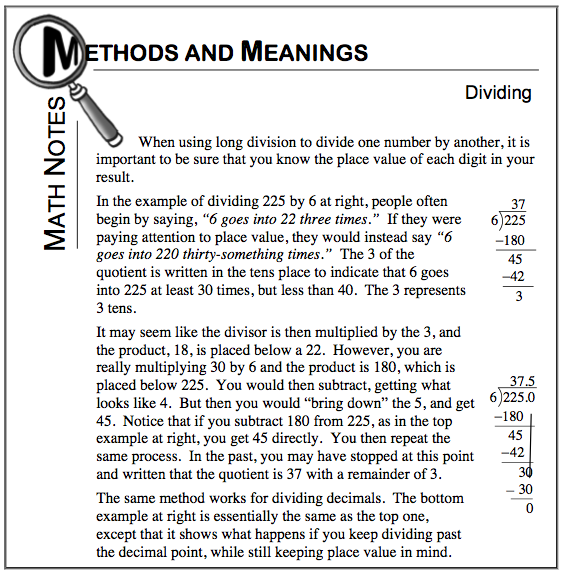### Home > CC1 > Chapter 4 > Lesson 4.2.2 > Problem4-62

4-62.

Find each quotient without using a calculator. Homework Help ✎

Refer to the Math Notes box at the bottom of the page (from Lesson 4.1.1) and problem 4-10 to review finding a quotient without a calculator.

1. $42.5\div1.5$

$28.\overline{3}$

1. $589.2\div16$

1. $5\div9$

$0.\overline{5}$